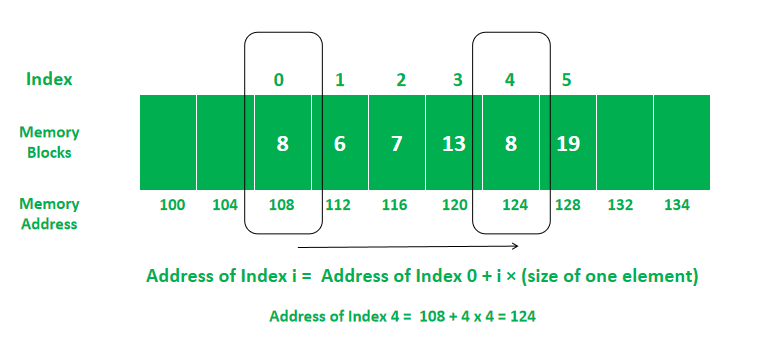# Why does accessing an Array element take O(1) time?

• Last Updated : 21 Jun, 2022

An array is a linear data structure. In an array, the operation to fetch a value takes constant time i.e., O(1).  Let us see why is it so.

Why the complexity of fetching value is O(1)?

As Arrays are allocated contiguously in memory, Fetching a value via an index of the array is an arithmetic operation. All arithmetic operations are done in constant time i.e., O(1).

Explanation:

In an array, we have the memory address of index 0 (Base address). By adding the product of the index number (of value to be fetched) and the size of one element (ex. int size is 4 bytes) with the base address, we can have the address of that index’s value. we don’t have to iterate through the array. So it’s done in O(1).

Address of ith Index = Base address + offset = Address of 0th Index + i × (size of one element)

Example:Memory Allocation In Array

In array A[] = {8, 6, 7, 13, 8, 19}

To fetch the value at index 4, we need the memory address where the value of that index is stored. The address can be obtained by doing an arithmetic operation i.e.

Address of value at index 4 = Address of index 0’s value + 4 × size of int = 108 + 4 × 4 bytes
Address of value at index 4 = 124
A = value at address 124 = 8

My Personal Notes arrow_drop_up
Recommended Articles
Page :